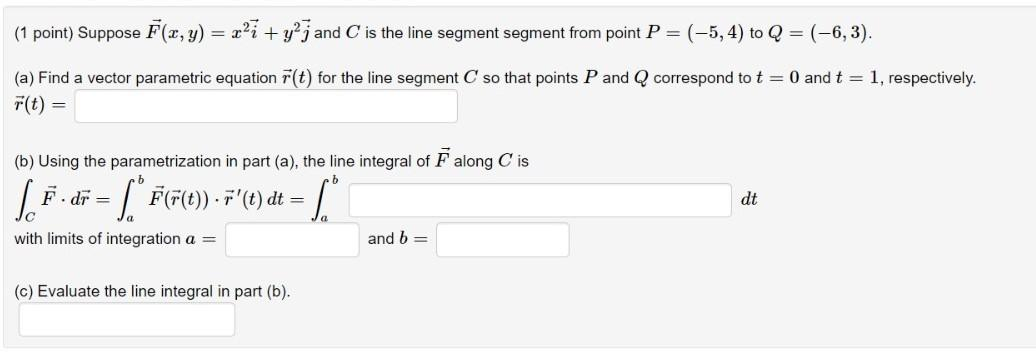### Create an Account

Already have account?

### Forgot Your Password ?

Home / Questions / (1 point) Suppose F(x, y) = r?ī + y²j and C is the line segment segment from point P=(-5, ...

# (1 point) Suppose F(x, y) = r?ī + y²j and C is the line segment segment from point P=(-5, 4) to Q = (-6,3). (a) Find a vector parametric equation F(t) for the line segment C so that points P and Q cor

(1 point) Suppose F(x, y) = r?ī + y²j and C is the line segment segment from point P=(-5, 4) to Q = (-6,3). (a) Find a vector parametric equation F(t) for the line segment C so that points P and Q correspond to t = 0 and t = 1, respectively. F(t) = (b) Using the parametrization in part (a), the line integral of F along Cis 1.5.de - [FF6). F'leydt - 1 dt with limits of integration a = and b= (c) Evaluate the line integral in part (b).Apr 14 2021 View more View Less

#### Answer (Solved)Subscribe To Get Solution